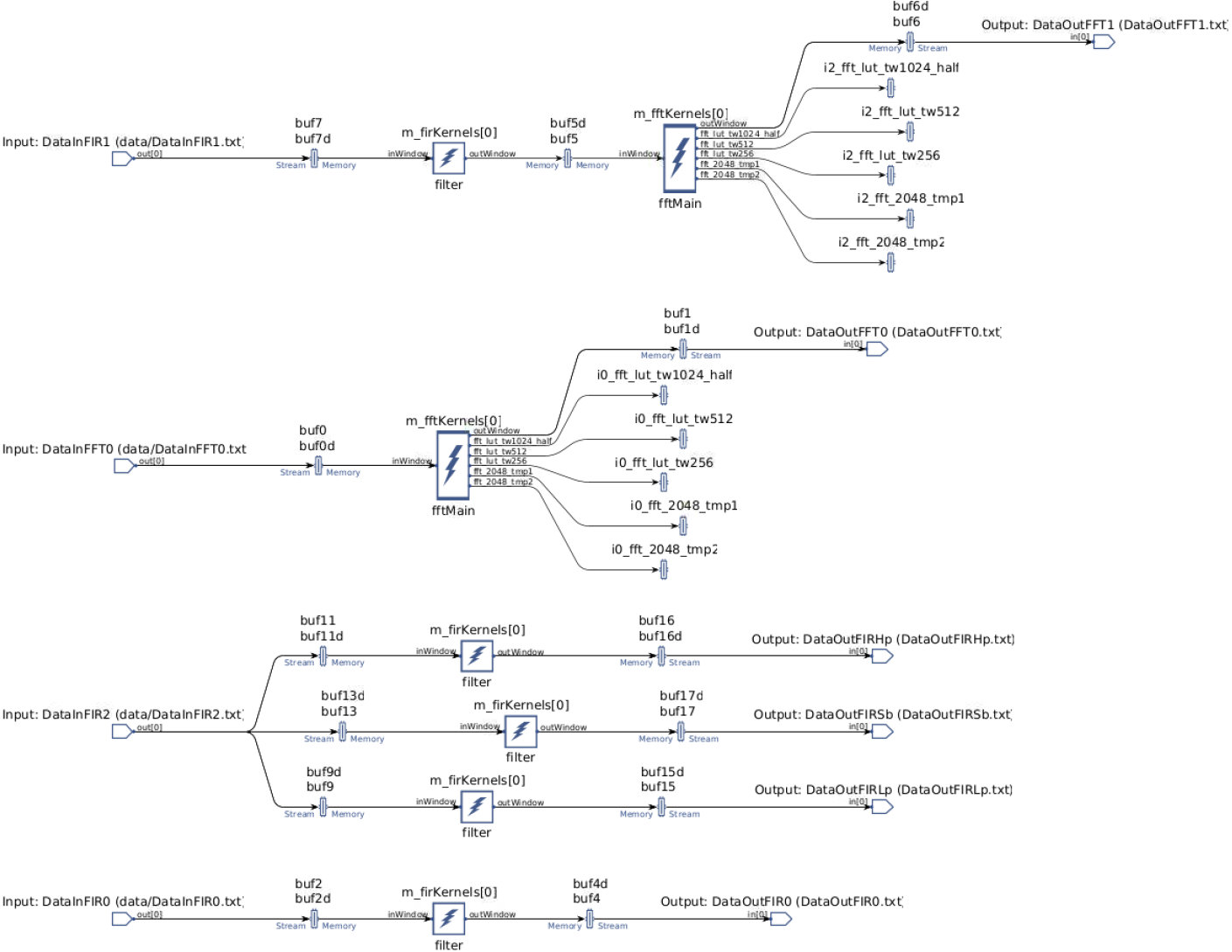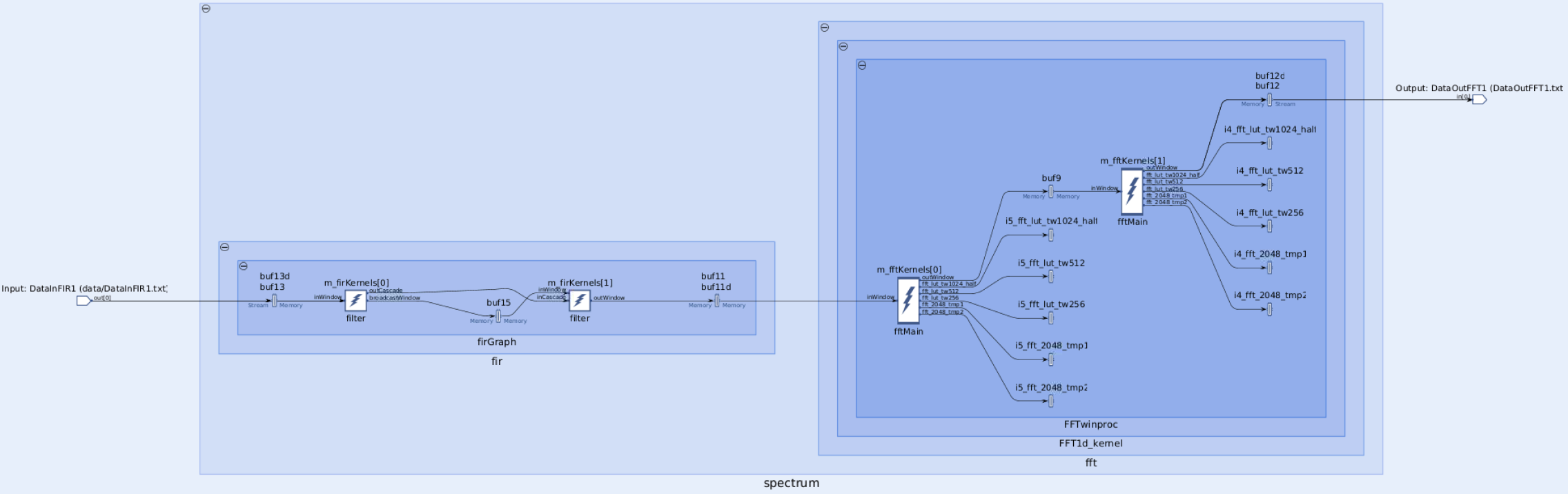# DSP Library Lab

## Goal

• Use the DSP Vitis Libraries for the AI Engine

## Design Overview

### AI Engine Kernels

For the AI Engine kernel we will use the FFT/iFFT as well as the single rate, symmetrical FIR filter from the DSP Libraries.

The templated parameters for the 1D FFT are defined here

The templated parameters for the symmetrical FIR filter are defined here

## Steps

This lab will make use of Makefile files to automate the building process.

### AIE Engine Graph

The FFT kernel depends in the Vitis Library repository.

``````cd \$HOME/xup_aie_training/
git submodule init && git submodule update
``````
2. Navigate to the `\$HOME/xup_aie_training/sources/dsplib_lab/aie` folder and run make

``````cd \$HOME/xup_aie_training/sources/dsplib_lab/aie
make
``````

The `aiecompiler` will be called to generate the `libadf.a` file. This process takes around 5 minutes.

Note: you can run `make -n` to see what command will be run without actually executing anything

3. Analyze the compilation results

``````vitis_analyzer build.hw/work/graph.aiecompile_summary
``````
4. In Vitis Analyzer, open the Graph tabNote that the Flat view is used

5. Questions for the reader by just looking at the information on Vitis Analyzer

• Q1: How many `input_plio` does the graph have?

• Q2: How many `output_plio` does the graph have?

• Q3: How many instances of the `xf::dsp::aie::fir::sr_sym::fir_sr_sym_graph` does the graph have?

• Q4: How many instances of the `xf::dsp::aie::fft::dit_1ch::fft_ifft_dit_1ch_graph` does the graph have?

• Q5: One `input_plio` port is broadcasted, how many kernels are connected to it?

• Q6: How many subgraphs are within the main graph?

• Q7: How many Tiles are used for AIE Kernels?

• Q8: How many Tiles are used for Buffers?

• Q9: How many Tiles are used for Stream Interconnect?

6. Explore the files in the directory `\$HOME/xup_aie_training/sources/dsplib_lab/aie/src/`

• `fft.hpp` declares a graph `FFT1d_graph` which instantiates the FFT function `xf::dsp::aie::fft::dit_1ch::fft_ifft_dit_1ch_graph`

• `fir.hpp` declares a graph `FIR_129_sym` which instantiates the FIR function `xf::dsp::aie::fir::sr_sym::fir_sr_sym_graph` it also declares four different set of taps. Note as we are using a symmetrical filter we only need to declare half of the coefficients plus the central tap

• `graph.hpp` declares the main graph `DSPLibGraph`. There is also the declaration of `SpectrumGraph`, which connects an fir with an fft instance. Finally, the declaration of `MultiFIRGraph` which instantiates three `FIR_129_sym` graph an initializes the tap values via an initialization list

• `graph.cpp` defines the emulation environment

In an AMD preconfigured instance you can run `code \$HOME/xup_aie_training/sources/dsplib_lab/aie/src/` to open the files with VS Code

### Run AIE Emulation

1. Run the AIE Emulation by executing the following code on the command line:

``````make aieemu
``````

This AIE emulation takes around 3 minutes.

Note: the AIE output results are not being checked

2. Visualize the emulation reports by running

``````vitis_analyzer build.hw/aiesimulator_output/default.aierun_summary
``````
3. Questions for the reader by just looking at the information on Vitis Analyzer

• Q10: How many cycle does one instance of the FIR takes to complete?

• Q11: How many cycle does one instance of the FTT takes to complete?

In this part we are going to modify the cascade length for both the FFT and FIR instances and see the changes in the graph and performance.

In an AMD preconfigured instance you can run `code \$HOME/xup_aie_training/sources/dsplib_lab/aie/src/` to open the files with VS Code

1. Open the file `\$HOME/xup_aie_training/sources/dsplib_lab/aie/src/fir.hpp` and change the macro `FIR129_CASCADE_LEN` to

``````#define FIR129_CASCADE_LEN 2
``````
2. Open the file `\$HOME/xup_aie_training/sources/dsplib_lab/aie/src/fft.hpp` and change the macro `FFT_CASCADE_LEN` to

``````#define FFT_CASCADE_LEN 2
``````
3. Save previous compilation results

``````cd \$HOME/xup_aie_training/sources/dsplib_lab/aie
``````
4. Recompile the AIE code

``````cd \$HOME/xup_aie_training/sources/dsplib_lab/aie
make
``````
5. Analyze the compilation results

``````vitis_analyzer build.hw/work/graph.aiecompile_summary
``````
6. In Vitis Analyzer, open the Graph tabNote only a portion of the graph is shown.

From this view, you can see that now the FIR instance is split in two kernels, which communicate using both a cascade interface and a buffer. Whereas, the FFT instance is also split in two kernels and these two kernels communicate using only a buffer.

### Run AIE Emulation for Cascaded Kernels

1. Run the AIE Emulation by executing the following code on the command line:

``````make aieemu
``````

This AIE emulation takes around 3 minutes.

Note: the AIE output results are not being checked

2. Visualize the emulation reports by running

``````vitis_analyzer build.hw/aiesimulator_output/default.aierun_summary
``````
3. Questions for the reader by just looking at the information on Vitis Analyzer

• Q12: How many cycle does one instance of the FIR takes to complete?

• Q13: How many cycle does one instance of the FTT takes to complete?

The following assignments are optional, however they will help deepen your knowledge about the DSP Libraries. No solution is provided for these assignments.

1. Use the Matrix Multiply from the DSPLib, add to the graph an instance of `xf::dsp::aie::blas::matrix_mult::matrix_mult_graph` using one of the real datatypes

Review the documentation of this library here

2. Implement a decimation symmetric FIR filter, add to the graph an instance of `dsplib::fir::decimate_sym::fir_decimate_sym_graph` using one of the real datatypes

Review the documentation of this library here

3. For the existing FIR filters use streaming interface instead of window interface

Review the documentation of this library here

4. Design your own single rate, asymmetrical filter.

For this, you will have to compute the coefficients and call the appropriate function

If you are attending an in-person tutorial, you can request support from your instructor. Otherwise, open a GitHub issue

## Appendix

• Q1: The graph has four `input_plio`

• Q2: The graph has six `output_plio`

• Q3: The graph has five `xf::dsp::aie::fir::sr_sym::fir_sr_sym_graph` instances

• Q4: The graph has two `xf::dsp::aie::fft::dit_1ch::fft_ifft_dit_1ch_graph` instances

• Q5: The input `input_plio` port `DataInFIR2` is broadcasted to three instances of `xf::dsp::aie::fir::sr_sym::fir_sr_sym_graph`

• Q6: There are two subgraphs: `SpectrumGraph` and `MultiFIRGraph`. The `fft` and `fir` graph are subgraph but not defined by our code

• Q7: 7 tiles are used for AIE Kernels

• Q8: 15 tiles are used for Buffers

• Q9: 19 Tiles are used for Stream Interconnect?

• Q10: Looking at the Profile tab, the function `filter` takes ~ 11,183 cycles

• Q11: Looking at the Profile tab, the function `fftMain` takes ~ 3,411 cycles

• Q12: With a cascade length of 2, the FIR is split into two kernels one `filter` instance takes ~ 7,336 cycles whereas the another takes ~ 8,7510 cycles. Bare in mind, that these two instances run in parallel

• Q13: With a cascade length of 2, the FFT is split into two kernels one `fftMain` instance takes ~ 1,785 cycles whereas the another takes ~ 1,657 cycles. Bare in mind, that these two instances run in parallel